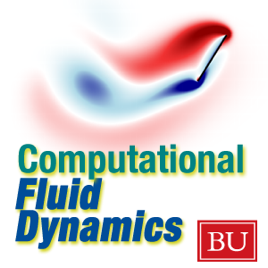6 episodes

There are many situations in which a computational approach is the ideal one for studying a problem. For example, in studying the flow of air in the breathing tract of a human, one can hardly do experiments! And even if we could experiment, how could we *see* what is happening? By simulation, we can both perform virtual experiments of the flow of fluids, and visualize the results in a myriad ways. Computational Fluid Dynamics (CFD) is vital for many applications of science and engineering. This course is designed for graduate students or advanced undergraduates.

Professor Lorena A Barba
Mechanical Engineering Department
Boston University# Computational Fluid Dynamics - ENG ME702 - Documents Boston University

• Technology

There are many situations in which a computational approach is the ideal one for studying a problem. For example, in studying the flow of air in the breathing tract of a human, one can hardly do experiments! And even if we could experiment, how could we *see* what is happening? By simulation, we can both perform virtual experiments of the flow of fluids, and visualize the results in a myriad ways. Computational Fluid Dynamics (CFD) is vital for many applications of science and engineering. This course is designed for graduate students or advanced undergraduates.

Professor Lorena A Barba
Mechanical Engineering Department
Boston University

ME702 course description

## ME702 course description

Lecture 0: Review of the differential form of the fluid equations

## Lecture 0: Review of the differential form of the fluid equations

Assignment 1: Computing the Navier-Stokes equations, steps 1 through 8

## Assignment 1: Computing the Navier-Stokes equations, steps 1 through 8

Assignment 2: Computing the Navier-Stokes equations, steps 9 to 12

## Assignment 2: Computing the Navier-Stokes equations, steps 9 to 12

Assignment 3: Burgers equation, with 5 schemes

## Assignment 3: Burgers equation, with 5 schemes

Assignment 4: Sod's test problems; use LF, LW, MacCormack and Richtmyer methods